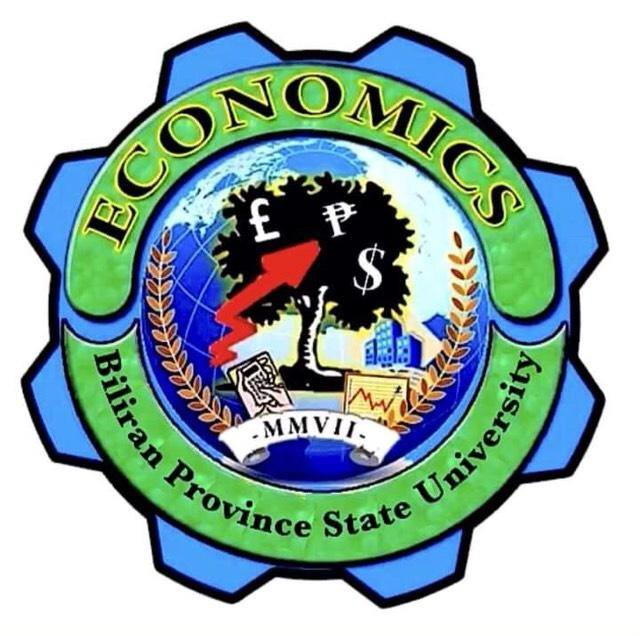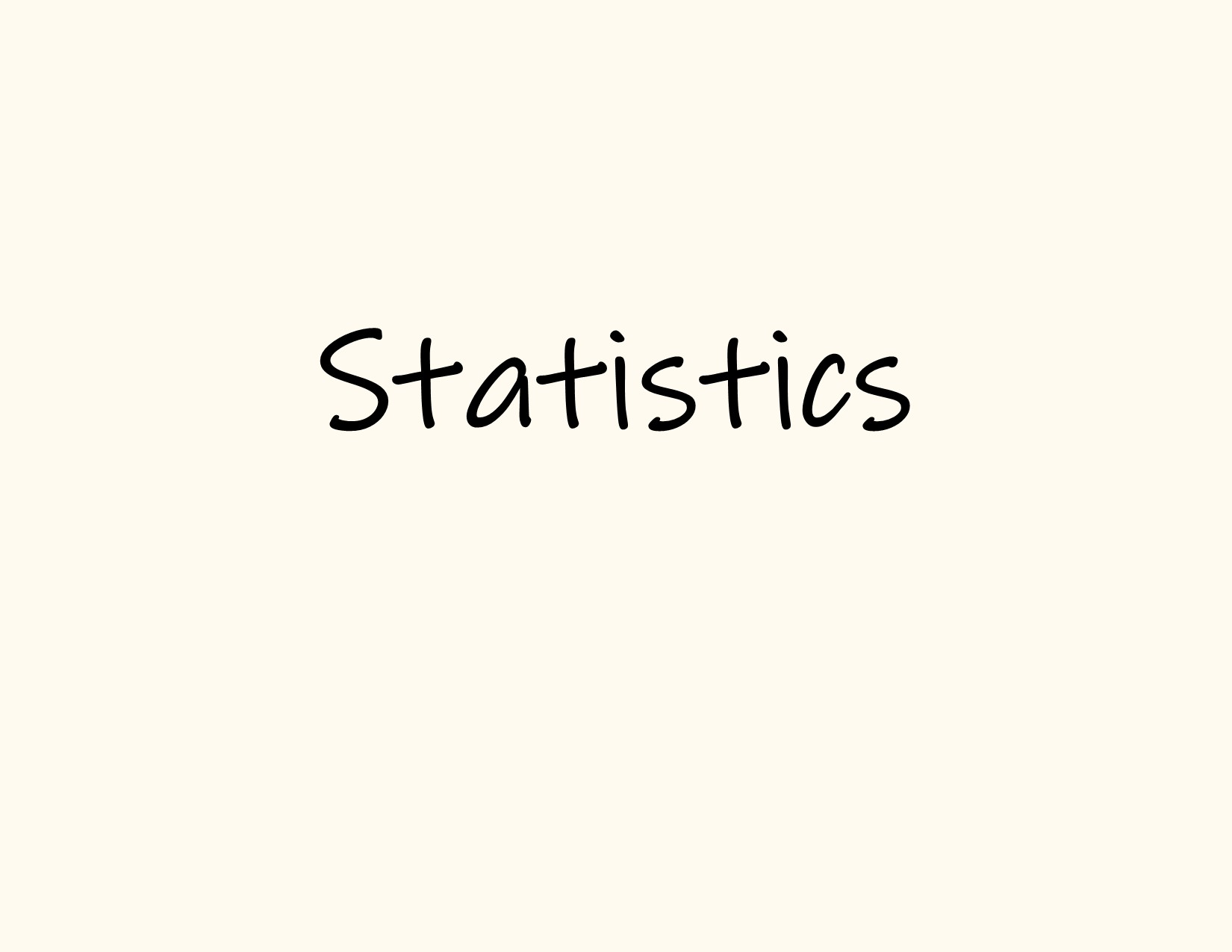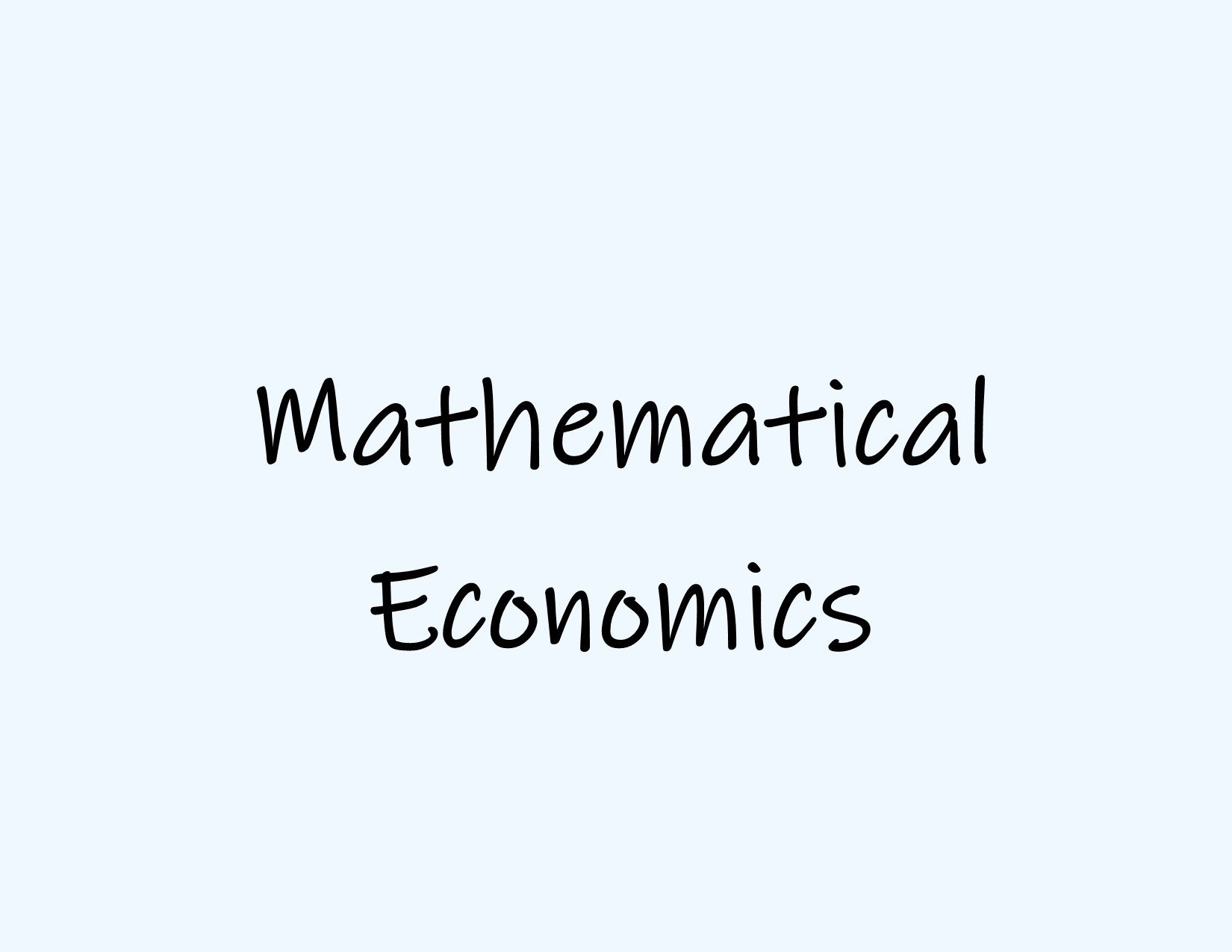### BA ECON 2A || 1ST SEM 2021-2022#### GE-RPH READINGS IN THE PHILIPPINE HISTORY || ECON 2A

Readings in the Philippine History is a three-unit lecture course offered to BA Economics students. The course is designed to research the local history and the current events in the economic situation in the country and demonstrate knowledge in the Philippine History and show skills in the selection of the learning resources, including ICT, and to create a historical mapping of their locality#### BC MATH 213 INTRODUCTION TO MATHEMATICAL STATISTICS || ECON 2A

Introduction to Mathematical Statistics is a 3-unit basic core course subject offered to BA Economics students. This is designed to give the students a background in basic statistical concepts. The study includes topics on collection and presentation of the different statistical data used in the field, frequency distribution, measures of central tendencies, measures of dispersion and location, normal distribution, and statistical tests and hypothesis testing. The students will compute and interpret numerical summaries and generate appropriate statistical tables and graphs based on the nature of variables measured and perform tests of significance concerning population mean and proportion for one and two populations.#### ECON 211 MATHEMATICAL ECONOMICS || ECON 2A

Mathematical Economics is a 3-unit basic core course subject offered to BA Economics students. This is designed to give the students the knowledge of the different mathematical techniques in the context of economics application that includes Algebra topics (i.e. algebraic expressions, graphs and functions, linear equations, quadratic equations), matrices, differentiation, and financial mathematics. The students will determine and calculate different economics concepts through Algebra computations, apply appropriate mathematical methods to solve problems involving the growth and decline over discrete time periods of some economic variables, solve a system of simultaneous equations through the use of matrices both manually and using a spreadsheet, and derive different economic concepts using differentiation.

The students are encouraged to write a data analysis paper integrating the different mathematical concepts and techniques in an economics context.